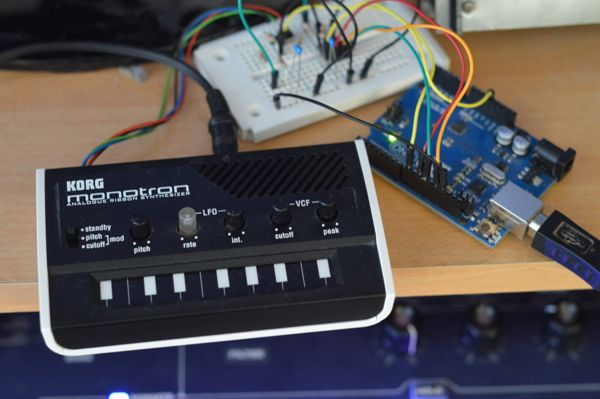## from now on you shalt be called miditron

As announced in my last post I have created a midi interface for my korg monotron using an arduino uno and an mcp4822 2 channel 12-Bit DAC.The DAC chip is controlled via the SPI and generates the control voltage for the pitch on channel 1 and the control voltage for the filter on channel 2. I used pin 8 of my arduino to controll the gate.

I got the Idea to midify my monotron from marc nostromo´s blog post - he uses a teensy 3.0 to create the midi-cv interface for the monotron. The SPI part of my code is from a blog post by Kerry D. Wong

To turn my arduino into a generic usb-midi device I used the hiduino library and the arduino midi library.

The filter cv is controlled using the mod-wheel in the HandleCC callback function. The Pitch gets changed every time a note on event is received. NoteOn events also set the gate to hight. NoteOff events only change the gate value if the pitch matches the last NoteOn event, all other NoteOff events are ignored, otherwise the sound would stop if overlapping keypresses are received.

To speed things up I used the PORTB technique to toggle the gate and I also turned of the midi-through functionality that is turned on by default. The values for the step-size and the tuning offset are the one that work for my monotron and will need some adjusting on other devices I also plan to add a variable resistor that makes the tuning of my miditron easier.

Next on my todo list is adding support for pitchbend, implementing an LFO for filter and pitch and soldering my circuit.

Here is the code that runs on my arduino:

``````#include <MIDI.h>
#include <SPI.h>

#define PB0 PORTB0

const int PIN_CS = 10;
const int GAIN_1 = 0x1;
const int GAIN_2 = 0x0;

float mtov;
int gate = LOW;
int ogate = LOW;
float volt = 0;
float filt = 0;
int ofs = 100.0;
int st = 95.0;

int lastnote = 0;

void setup() {

float s = 4096/st;
for(int i=0; i<120; i++) {
mtov[i] = i*s + ofs;
}

pinMode( 8, OUTPUT );
pinMode( 9, OUTPUT );

pinMode(PIN_CS, OUTPUT);
SPI.begin();
SPI.setClockDivider(SPI_CLOCK_DIV2);

MIDI.begin();
MIDI.turnThruOff();
// The latest Arduino MIDI Library (3.1) uses C style function pointers to create a callback system for handling input events.
MIDI.setHandleNoteOn(HandleNoteOn);
MIDI.setHandleControlChange(HandleCC);
MIDI.setHandleNoteOff(HandleNoteOff);
}

void loop() {
}

//assuming single channel, gain=2
void setOutput(unsigned int val) {
byte lowByte = val & 0xff;
byte highByte = ((val >> 8) & 0xff) | 0x10;

PORTB &= 0xfb;
SPI.transfer(highByte);
SPI.transfer(lowByte);
PORTB |= 0x4;
}

void setOutput(byte channel, byte gain, byte shutdown, unsigned int val) {
byte lowByte = val & 0xff;
byte highByte = ((val >> 8) & 0xff) | channel << 7 | gain << 5 | shutdown << 4;

PORTB &= 0xfb;
SPI.transfer(highByte);
SPI.transfer(lowByte);
PORTB |= 0x4;
}

void HandleNoteOn(byte channel, byte pitch, byte velocity) {
if (velocity == 0 ) {
HandleNoteOff( channel, pitch, velocity );
} else {
lastnote = pitch;
gate = HIGH;
volt = mtov[pitch-26];

setOutput(1, GAIN_2, 1, volt );

if ( gate != ogate ) {
ogate = gate;
if (gate == LOW) {
PORTB &= ~_BV(PB0);
} else {
PORTB |= _BV(PB0);
}
}
}
}

void HandleCC(byte channel, byte number, byte value) {
if ( number == 1 ) {
filt = value;
setOutput(0, GAIN_2, 1, filt * 32);
}
}

void HandleNoteOff(byte channel, byte pitch, byte velocity) {
// Do something here
if (pitch == lastnote) {
gate = LOW;

if ( gate != ogate ) {
ogate = gate;
if (gate == LOW) {
PORTB &= ~_BV(PB0);
} else {
PORTB |= _BV(PB0);
}
}
}
}
``````

Leave a response

1. Nice ! Happy you got inspired...

2. I'm the guy from twitter that can't get the MCP4822 only a DAC0800.

Thank you very much.

3. Do you think the Arduino'd still have enough memory to control two Monotrons (Pitch CV, gate, Midi synced filter Cutoff) off of the same setup (the MCP is a dual DAC)?

Cheers and thanks for sharing all of this.

4. I'm not sure if that works. The MCP4822 can provide 2 cv channels. In theory you could connect multiple spi devices to the arduino, but you cant control them simultanious. you have to switch between them and I'm not sure if you can do that fast enough. what you could try is to control the pitch of both monotrons with the two cv outs of the mcp and control the filter cv using a slower lowpass filtered analog out of the arduino

5. hello, where can I find the project's scheme? thank's for your job